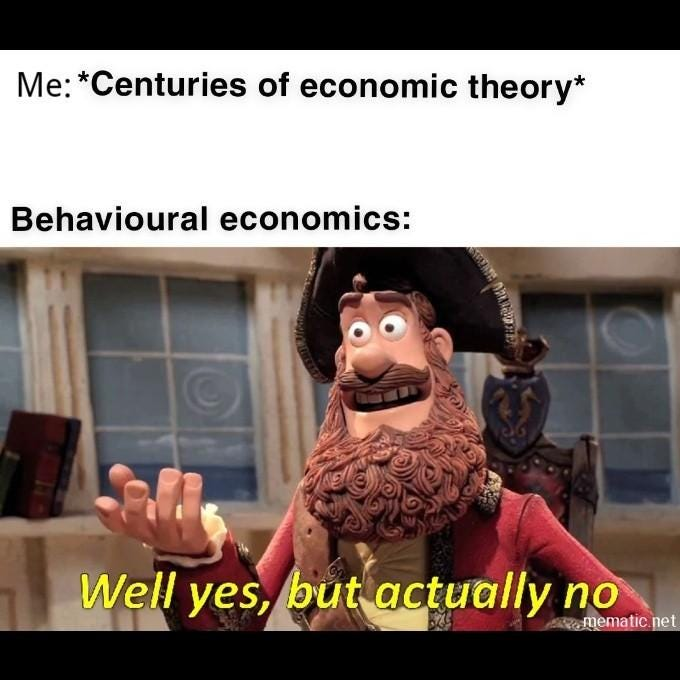# What is so hot about Behavioral Economics?

A quick and simple introduction to one of the hottest topics in the field of Economics.Taken from google. Whoever made this, you are awesome.

The field of Economics was once considered boring and mundane, but not anymore. The field has garnered quite the popularity thanks to the rise of Behavioral Economics - a revolutionary idea that started a long time ago and was popularised through excellent books such as thinking fast and slow by Daniel Kahneman as well as Nudge by Richard Thaler.

But what’s the big idea behind Behavioral Economics? Why is it popular among academics and non-academics alike? To understand the beauty, we must first embark on a journey to understand choice under uncertainty as well as rationality.

The field of Economics has evolved in a lot of ways since the times of Adam Smith. At its heart though, Economics tries to answer this one question:

Given a set of possible choices and uncertainty, how would one make a rational decision?

The word ‘rational’ is bolded on purpose. There is a need to define rationality as far as Economics is concerned, because well, rationality is a vague concept. Perhaps it is for this very reason Economics is called the dismal science. How can we create models for something that is so vague and abstract? This is quite different from pure mathematics where even though it is studying and analysing abstract concepts, there are underlying reliable rules that can be invented or found.

The great economists of the past however, thought of a brilliant idea. Instead of forcing our way through into analysing a very abstract concept, why don’t we transform the problem statement into something that is more predictable? Hence, the creation of a perfectly rational creature that makes decisions based on gains and losses alone: Homo Economicus. And the best thing about homo economicus is that his rationality follows six assumptions outlined below:

• Completeness
• Transitivity
• Monotonicity
• Continuity
• Reduction
• Substitution

While it is possible to talk about each assumption in great details, I shall leave it for the future. But instead let’s talk about the implication. Mathematically, the six assumptions above gave rise to Von neumann-Morgenstern (VnM) utility function given by the following equation:

The utility¹ of a lottery² is equal to the expected utility of the individual prizes, which implies one’s preference is linear with probabilities. So how does this equation relate to rationality and decision making? According to the equation, a rational human will make a decision in such a way that it will maximise his utility. In other words, a rational human being is a utility maximizer. Additionally he only cares about the absolute value of the final outcome.

This means that before a homo economicus makes a decision, he will analyse the possible gains and losses objectively and perfectly. And also, he will always be consistent with his decisions. The problem is we are not a homo economicus. We cannot be objective and consistent all the time. For example, we might hate one thing when we were children but love that very thing we hated as we grow older (broccoli in my case). Additionally, we are susceptible to cognitive biases. One famous example will be the disposition effect³. For the geeks out there, it is also possible to construct simple mathematical examples to violate the axioms above (see Allais’ paradox for more details).

Lucky for us, the field of Psychology has conducted a lot of experiments on human behavior and found out that a normal human being (unless you are Spock) is susceptible to at least one of the following:

• Framing Effect
• Endowment Effect
• Loss Aversion
• Poor estimator of probabilities

Framing effect simply means we are susceptible to well, framing. One example will be the FOMO syndrome that is prevalent in social media. Endowment effect means that it is difficult to part with something that we already own. Loss aversion explains why losing £1000 affects us more than gaining £1000. Last but not least, we are just extremely bad at estimating probabilities. In the casino for example, we tend to overestimate our probability of winning even though it is actually quite low.

So the question is, can we find a simple equation that takes into account the 4 things above to model how we make decisions under uncertainty? And the answer is, yes we can. Thanks to Daniel Kahneman and Amos Tversky who developed the prospect theory. The prospect theory takes into account the 4 biases above by modifying the expected utility function into the following:

The first term of the equation is a probability weighting function that takes into account the fact that we are very poor at estimating probabilities. The second term is a value function that takes into account framing effect, endowment effect and loss aversion. Voila! Suddenly we have this simple yet powerful equation that gives insights on how we make decisions under uncertainty without forcing us to be a homo economicus; a being that is not susceptible to biases and devoid of any emotions. And that what is so hot about behavioral economics.

: Utility is a convenient word that economists use to describe both material and not material things such as money, happiness, satisfaction, etc.

: Economists like to use the word lottery well because it conveys the message that there are risks and uncertainty involved.

: Disposition effect refers to investors reluctance to sell assets that have lost value and greater likelihood of selling assets that have gained value.

A full-time nerd